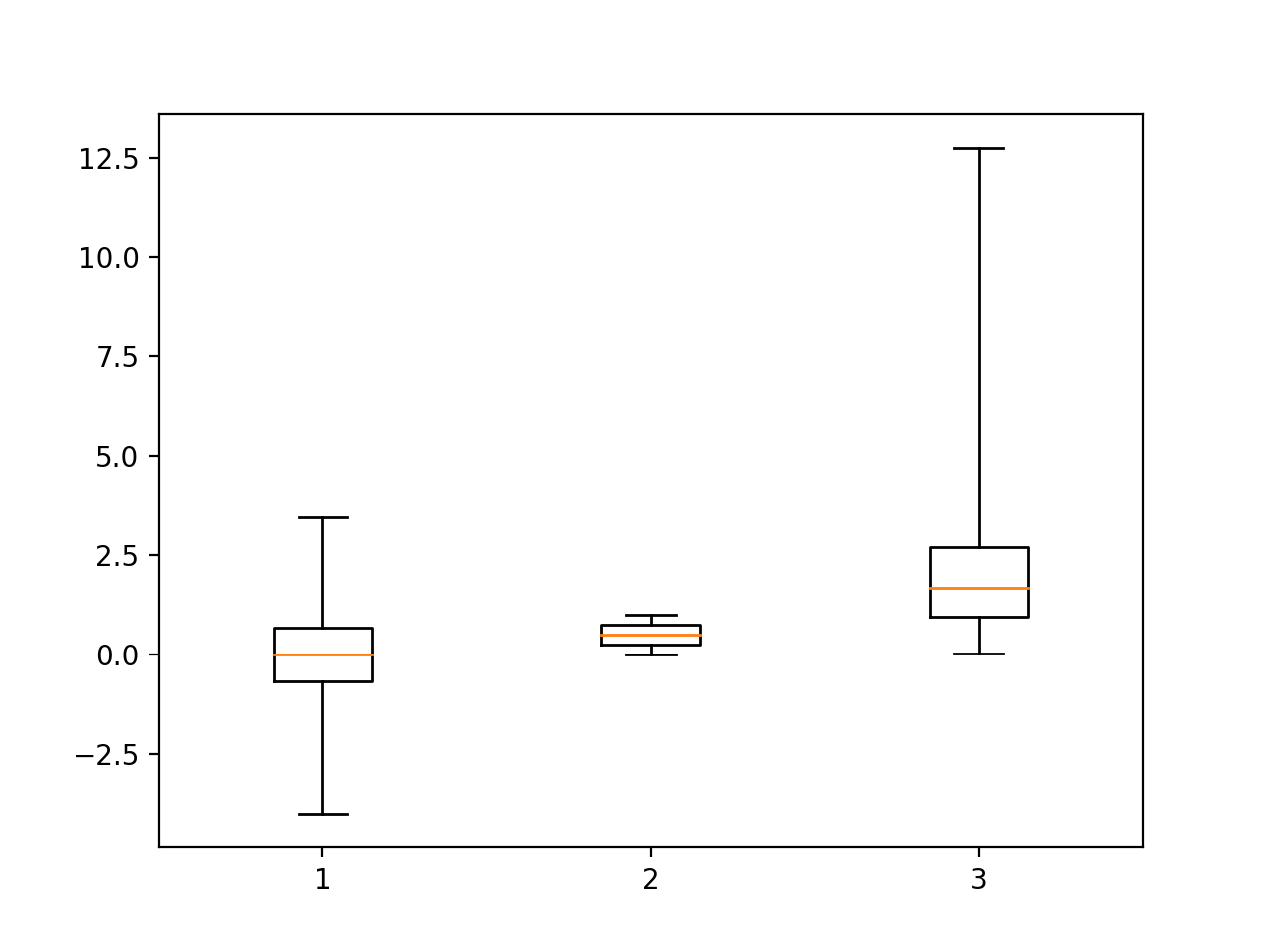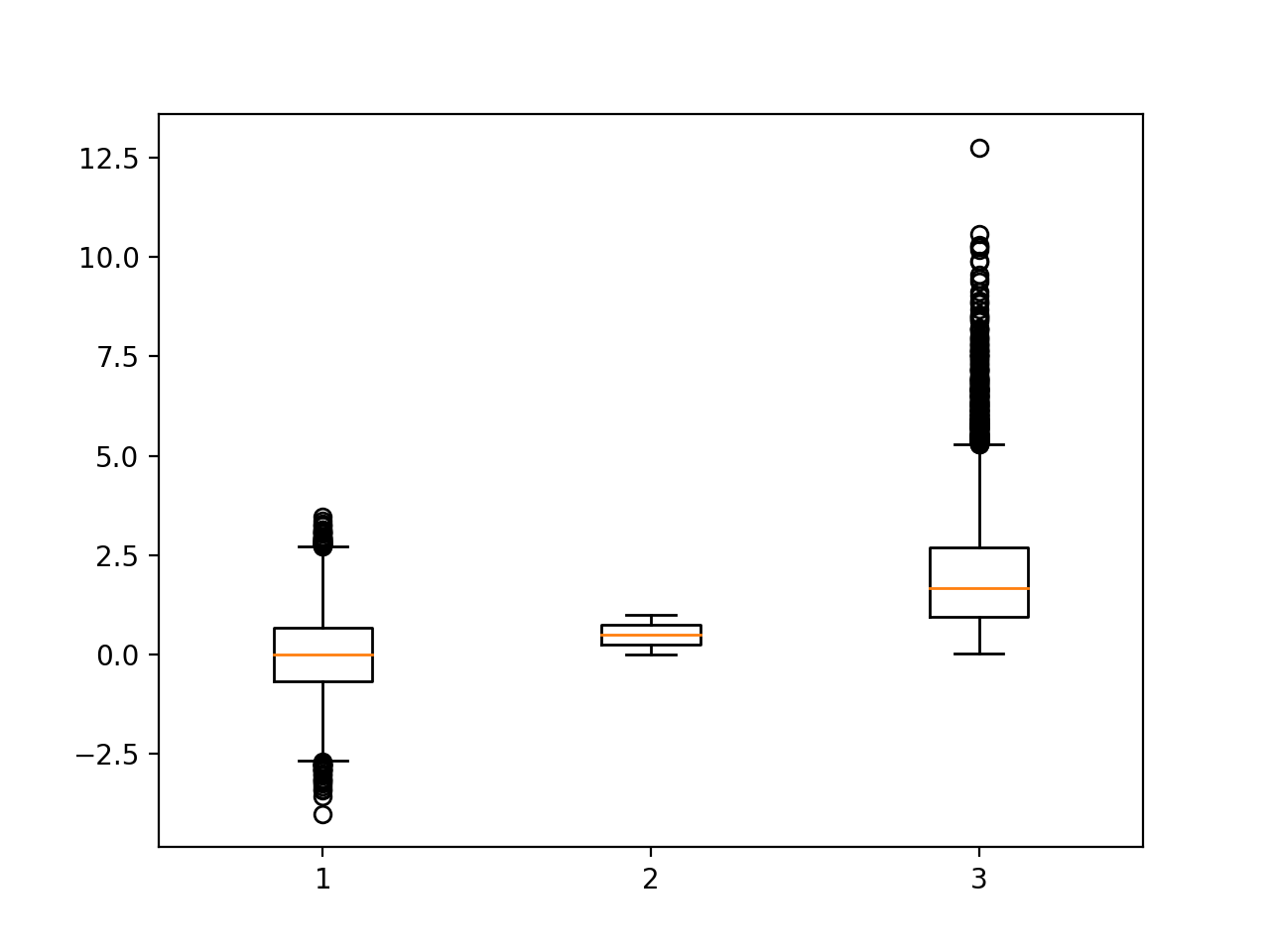# Box Plots

A box plot (or, box and whisker plot) is a method of showing aggregate statistics of various samples in a concise manner. For each sample, it simultaneously shows:

• median of each sample,
• minimum and maximum of the samples, and the
• interquartile range.

The following code block creates three different samplings from numpy. A normal distribution, a random distribution, and a gamma distribution.

%matplotlib notebook

import pandas as pd
import numpy as np
import matplotlib.pyplot as plt

normal_sample = np.random.normal(loc=0.0, scale=1.0, size=10000)
random_sample = np.random.random(size=10000)
gamma_sample = np.random.gamma(2, size=10000)

df = pd.DataFrame({'normal': normal_sample,
'random': random_sample,
'gamma': gamma_sample})

df.describe()


normal random gamma
count 10000.000000 10000.000000 10000.000000
mean 0.005574 0.503947 1.980398
std 1.003427 0.289082 1.400785
min -4.013316 0.000017 0.014506
25% -0.666291 0.252406 0.947084
50% -0.004336 0.507529 1.671177
75% 0.684656 0.751771 2.687132
max 3.474749 0.999931 12.756334

The summary statistics shown above require splitting the data into four quarters. The first quarter is between the minimal value and the first 25% of the data. That first 25% is called the first quartile. The seconda nd third quarters of the data are between the first quartile and the 75% mark, which is called the third quartile. The finnal quarter of the data is between the third quartile and the maximum.

The interquartile range is between the first and third quartiles.

In the box plots shown below the:

• interquartile range is the boxed region, the
• maximum and minimum values are the horizontal lines, and the
• median is the red line in the center of the boxed region.

It is also possible to specify more limited ranges for the whiskers. This is changeable via the whis parameter to the function. The ‘range’ parameter means use the maximum and minimum of the data.

plt.figure()

plt.boxplot([ df['normal'],
df['random'],
df['gamma'] ],
whis='range');

<IPython.core.display.Javascript object>If the whis parameter is left out, the top whisker defaults to reaching to the last datum less than Q3 + 1.5*IQR, where IQR represents the interquartile range.

Plots like the one shown below are good for detecting outliers. The datapoints beyond the whiskers are called “fliers.”

plt.figure()

plt.boxplot([ df['normal'],
df['random'],
df['gamma'] ]);

<IPython.core.display.Javascript object>